二、标准差 《医学统计学》

# 二、标准差

1．标准差的公式　样本标准差是用得最多的变异指标，其公式为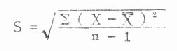(4.14)

2．标准差的计算

（1）按基本公式（4.14）计算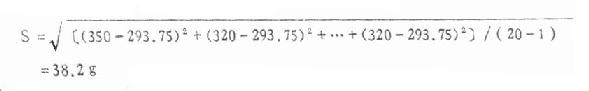（2）递推法当用电子计算机进行计算，希望每输入一个数据，都能得到X与S，则将式（4.8）与式

（4.5）配合计算。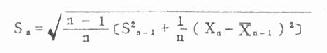(4.15)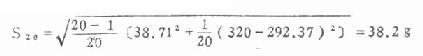（3）直接法 不需先计算均数，直接用变量值代入式（4.16）或式(1.17)计算。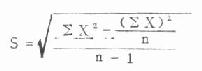（4.16)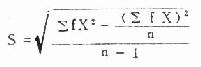(4.17)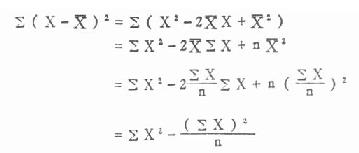∑X=2+3+3+…+6+8=45

∑X2=22+32+32+…62+82=229

∑fX=1×2+2×3+…+1×6+1×8=45

∑fX2=1×22+2×32+…1×62+1×82=229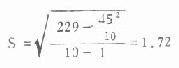上一页：一、变异指标的意义及种类 当前页：二、标准差 下一页：三、变异系数